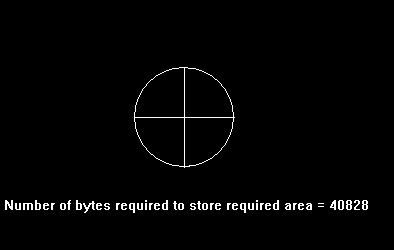# imagesize() function in C

• Difficulty Level : Medium
• Last Updated : 23 Jan, 2018

The header file graphics.h contains imagesize() function which returns the number of bytes required to store a bit-image.
Syntax :

```unsigned int imagesize(int left, int top,
int right, int bottom);

where,
left, top, right, and bottom
define the area of the screen
in which image is stored.
```

Below is the implementation of imagesize() function.

 `// C Implementation for imagesize()``#include ``#include `` ` `// driver code``int` `main()``{``    ``// gm is Graphics mode which is``    ``// a computer display mode that``    ``// generates image using pixels.``    ``// DETECT is a macro defined in``    ``// "graphics.h" header file``    ``int` `gd = DETECT, gm, color, bytes;``    ``char` `arr;`` ` `    ``// initgraph initializes the``    ``// graphics system by loading a``    ``// graphics driver from disk``    ``initgraph(&gd, &gm, ``""``);`` ` `    ``// Draws a circle with center at``    ``// (200, 200) and radius as 50.``    ``circle(200, 200, 50);`` ` `    ``// draws a line with 2 points``    ``line(150, 200, 250, 200);`` ` `    ``// draws a line with 2 points``    ``line(200, 150, 200, 250);`` ` `    ``// imagesize function``    ``bytes = imagesize(150, 150, 250, 250);`` ` `    ``// sprintf stands for “String print”.``    ``// Instead of printing on console,``    ``// it store output on char buffer``    ``// which are specified in sprintf``    ``sprintf``(arr, ``"Number of bytes required "``         ``"to store required area = %d"``, bytes);`` ` `    ``// outtext function displays text``    ``// at current position.``    ``outtextxy(20, 280, arr);`` ` `    ``getch();`` ` `    ``// closegraph function closes the``    ``// graphics mode and deallocates``    ``// all memory allocated by``    ``// graphics system .``    ``closegraph();`` ` `    ``return` `0;``}`

Output :

``````

My Personal Notes arrow_drop_up